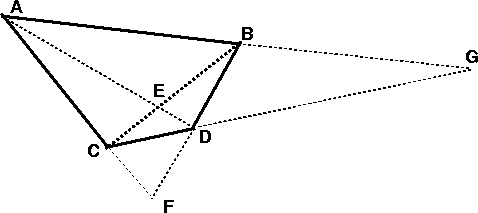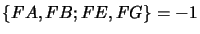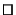Next: Involutions Up: Harmonic Ratios and Involutions Previous: Definition

Any set of four non-aligned points A,B,C,D in the plane can be joined pairwise by six distinct lines. This figure is called the complete quadrangle. The intersections of opposite sides (including the two diagonals) produce three further points E,F,G (see fig. 3.4).Property: The pencil of four lines based at each intersection of opposite sides is harmonic. For example.

A simple proof maps the quadrangle projectively onto a rectangle, sending F and G to points at infinity in two orthogonal directions. The four lines of the pencil become parallel, with one being at infinity. The line through E is obviously half way between those through A and B, so by the previous exercise the line configuration is harmonic.Exercise 3.6   : Given the images a,b,c of two points A,B on a 3D line and its 3D vanishing point (point at infinity) C, construct the projection of the mid-point of AB using just a ruler.

Bill Triggs
1998-11-13